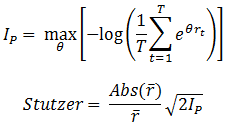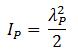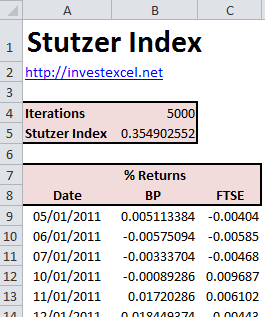Stutzer Index

This article introduces the Stutzer index and provides a calculation spreadsheet. The Stutzer index is an investment performance benchmark that penalizes underperformance against a benchmark.

It is based on the assumption that fund managers will favor portfolios whose probability of negative returns over a benchmark decays to zero  over the long term. Its characteristics make it particularly suitable for benchmarking the performance of hedge funds (because it does not assume normally distributed returns) and pension funds (because of its suitability for long-term investments).

The Stutzer index is simply the speed of decay (with a higher value being better) , and is defined by the following equations• rt are the excess returns
• IP is the information statistic
• θ is a numerically calculated parameter than maximizes IP
• r-bar is the average excess return

When the returns are normally distributed, the Stutzer Index and the Sharpe Ratio are equal, as indicated by this equation (where λP is the Sharpe Ratio)This approach has two major advantages

• It penalizes negative skew and high kurtosis, hence favoring portfolios with favorible returns distributions
• Rankings are consistent with the Sharpe Ratio as long as the returns are normally distributed
However, the Stutzer index has several dissadvantages
• It is more difficult to compute than the Sharpe Ratio
• Long time periods are required for rigorous results
• Investors’ aversion to risk reduces as their wealth increases; hence the assumption of constant risk aversion is not necessarily valid

Calculate the Stutzer Index in Excel